## Definition

### For a function of two variables

Suppose$F$ is a function of two variables$x$ and$y$. We say that$F$ is additively separable if there exist functions$f,g$ of one variable such that:$F(x,y) = f(x) + g(y)$

on the entire domain of$F$.

Note that the concept of additively separable is sensitive to the coordinate system, i.e., if we change the coordinate system, a function that was originally additively separable need not remain additively separable.

### For a function of many variables

Suppose$F$ is a function of$n$ variables$x_1,x_2,\dots,x_n$. We say that$F$ is completely additively separable if there exist functions$f_1,f_2,\dots,f_n$, each a function of one variable, such that: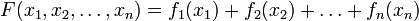$F(x_1,x_2,\dots,x_n) = f_1(x_1) + f_2(x_2) + \dots + f_n(x_n)$

(note that the subscripts here are not to be confused with subscripts used for partial derivatives).

There is a weaker notion of partially additively separable: if we express the set$\{ 1,2,\dots,n\}$ as a union of two disjoint subsets$A,B$,$F$ is additively separable with respect to the partition if there exist functions$f_A,f_B$ such that:$F(x_1,x_2,\dots,x_n) = f_A(\mbox{only the variables } x_i, i \in A) + f_B(\mbox{only the variables } x_i, i \in B)$

## Partial derivatives

Additively separable functions are the exceptions to the general rule that value of partial derivative depends on all inputs.

Version type Statement about first-order partial derivatives Statement about second-order mixed partial derivatives
additively separable function$F$ of two variables$x,y$, both pieces are differentiable functions, written as$F(x,y) = f(x) + g(y)$$F_x(x,y) = f'(x)$ (independent of$y$)$F_y(x,y) = g'(y)$ (independent of$x$)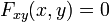$F_{xy}(x,y) = 0$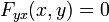$F_{yx}(x,y) = 0$
completely additively separable function$F$ of$n$ variables$x_1,x_2,\dots,x_n$, written as$f_1(x_1) + \dots + f_n(x_n)$$F_{x_i}(x_1,x_2,\dots,x_n) = f_i'(x_i)$ for each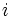$i$. Note that each first-order partial depends only on that variable and not on the others.$F_{x_ix_j}(x_1,x_2,\dots,x_n) = 0$ for each$i,j$.
partially additively separable function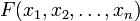$F(x_1,x_2,\dots,x_n)$ equals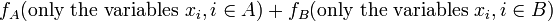$f_A(\mbox{only the variables } x_i, i \in A) + f_B(\mbox{only the variables } x_i, i \in B)$ Each first-order partial of$F$ with respect to a variable in$A$ equals the corresponding first-order partial of$f_A$, and in particular depends only on the variables within$A$.
Each first-order partial of$F$ with respect to a variable in$B$ equals the corresponding first-order partial of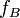$f_B$, and in particular depends only on the variables within$B$.
Any second-order mixed partial involving a variable in$A$ and a variable in$B$ is zero.